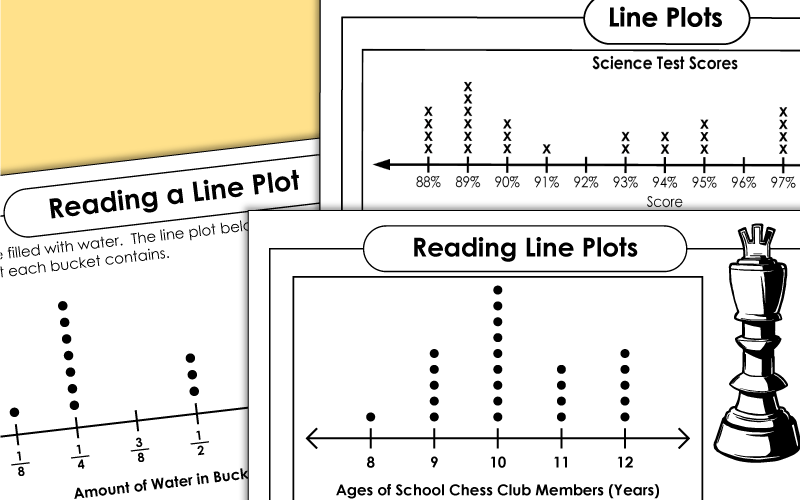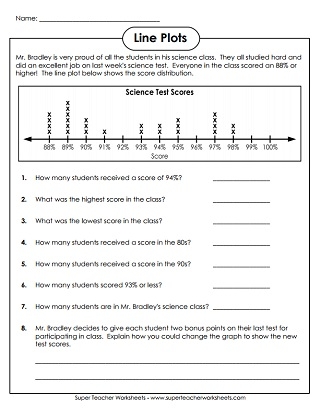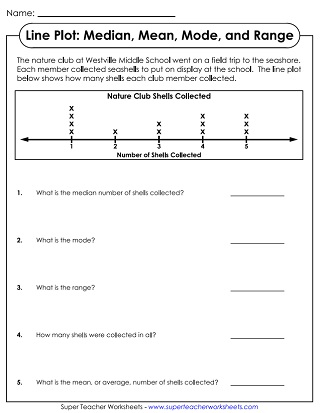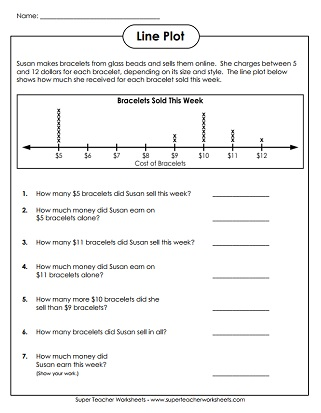# Line Plots

This page contains worksheets with line plots, a type of graph that shows frequency of data along a number line.## Basic Line Plots

The line plot shows the scores students received on a science test.  Use the data on the graph to answer the questions. Grade 3 and up.
This line plot data shows how many bracelets Susan sold.  Use the data on the line plot to answer the questions. Grade 3 and up.
A music teacher gives her class a 6-question test about composers.  Show her students' scores on a line plot. Grade 3 and up.
This 3-page worksheet packet will have students interpret data, create two line plots, and answer questions.
This line plot (dot plot) shows ages of chess club members. Answer the questions using the plot/graph.
Jeremy's grandfather gave him a dozen wheat pennies.  Make a line plot to show the dates on the pennies. Grade 3 and up.
This page has two line plots. Students use the data on the graphs to answer the questions.
The line plot shows the number of points each student earned in a social studies review game.  Answer the questions that follow. Grade 3 and up.

Plot the mean, median, mode, and range of the data shown on the line plot.  Grades 4 and up.
Make a line plot to show how many of each jar are in a set. This activity requires students to have a basic understanding of capacity measurement. They'll need to convert quarts and pints into fractional units of a gallon. (example: 1 quart = 1/4 of a gallon)
A line plot shows the amount of water in 17 buckets. This lessons requires knowledge of standard units of capacity (gallons, quarts, and pints).

Pictograph Worksheets

Pictographs use symbols to show data.

Line Graph Worksheets

Line graphs (not to be confused with line plots) have plotted points connected by straight lines.

Pie Graph Worksheets

Pie graphs, or circle graphs, show percentages or fractions of a whole group.

Bar Graphs

Students can practice creating and interpreting bar graphs on these printable worksheets.

## Sample Worksheet ImagesMy Account
Site Information